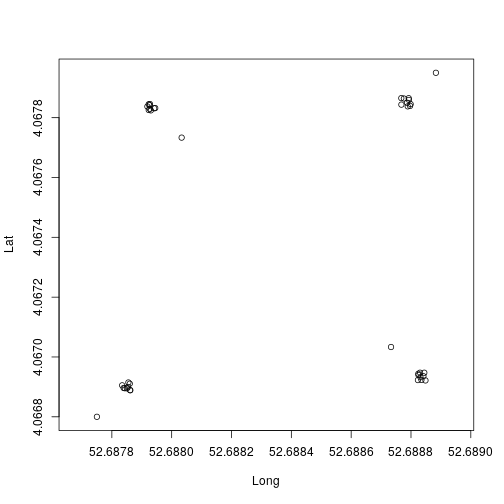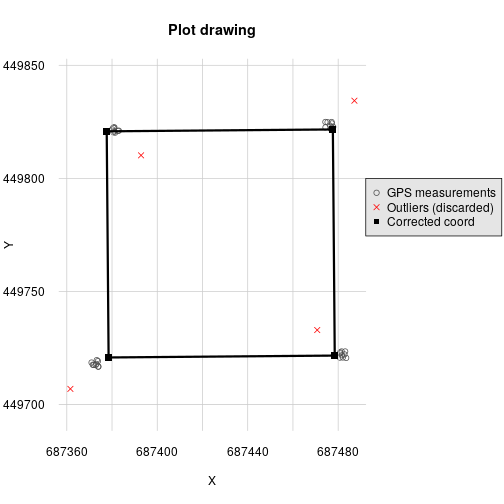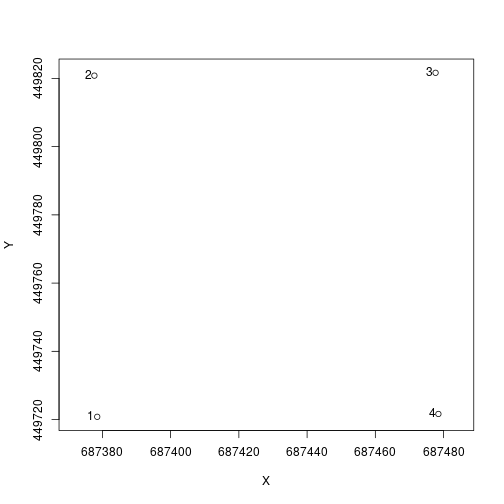# Requirement

You need the following information to use the following function in your analysis :

• Dimension of the plots
• Coordinate GPS of minimum 3 points non-aligned and relatily far away with the corresponding relative coordinates
• The origin corner
• The X, Y directions
``````trees <- read.csv(system.file("external", "NouraguesPlot.csv",
package = "BIOMASS", mustWork = T
))
``````

Table: Head of the table trees

plot xRel yRel D WD H
NB1 1.30 4.7 11.459 0.643 12
NB1 2.65 4.3 11.618 0.580 16
NB1 4.20 6.9 83.875 0.591 40
NB1 5.90 4.7 14.961 0.568 18
NB1 6.40 4.1 36.765 0.530 27
NB1 13.50 2.3 13.528 0.409 20

We can see in the table that we have for each trees the name of the plot we have, the `xRel` and `yRel`, the relative coordinate of the trees inside the plot. The rest of the column is for calculate the AGB at the end.

``````coord <- read.csv(system.file("external", "Coord.csv",
package = "BIOMASS", mustWork = T
))

plot(coord[, c("Long", "Lat")], asp = 1)
``````We can see on the plot that the corner coordinates are spread.

Table: Head of the table coord

Plot Corners Lat Long xRel yRel
NB1 NB1_SW 4.067 52.689 0 0
NB1 NB1_SW 4.067 52.689 0 0
NB1 NB1_SW 4.067 52.689 0 0
NB1 NB1_SW 4.067 52.689 0 0
NB1 NB1_SW 4.067 52.689 0 0
NB1 NB1_SW 4.067 52.689 0 0

In the table, we have the name of the plot, the coordinate `Lat`, `Long` (or another projected coordinates), and `xRel`, `yRel`, the relative coordinate for the points observed.

# To manage the plots

## Import the dataset and visualisation

The plot is referenced in the longitude latitude coordinate so you must have the package `proj4` if you are in this situation. If you have projected coordinate, you can continue with the `projCoord` argument instead of `longlat` argument.

## Correct the GPS coordinate

``````correct_plot <- correctCoordGPS(
longlat = coord[, c("Long", "Lat")],
coordRel = coord[, c("xRel", "yRel")],
rangeX = c(0, 100), rangeY = c(0, 100), drawPlot = T,
maxDist = 10, rmOutliers = T
)
````````````
str(correct_plot, max.level = 1)
#> List of 5
#>  \$ cornerCoords  :'data.frame':  4 obs. of  2 variables:
#>  \$ correctedCoord:'data.frame':  40 obs. of  2 variables:
#>  \$ polygon       :Formal class 'SpatialPolygons' [package "sp"] with 4 slots
#>  \$ outliers      : int [1:4] 10 20 30 40
#>  \$ codeUTM       : chr "+proj=utm +zone=39 +north +ellps=WGS84 +datum=WGS84 +units=m +no_defs"
``````

The output of the function is a list with a data.frame `corner` it's the corner of the plot, `polygon` the spatial polygon, `outliers` the vector with the line number of the outliers and `codeUTM` the UTM code for the polygon.

The outliers are calculated by a measure of distance between the predicted points and the GPS points. If this distance is higher than the value of `maxDist`, the point is considered like outliers.

## Numbering the corner

We have to number the corner of the plot, it is working if we have exactly 4 points for each plot, so we have to do the correctCoordGPS before if we have not the correct number of points.

``````coord_num <- numberCorner(
projCoord = correct_plot\$cornerCoords,
plot = rep("NB1", 4),
origin = c(F, F, F, T),
clockWise = T
)

plot(coord_num[, c("X", "Y")], asp = 1)
text(coord_num[, c("X", "Y")], labels = coord_num[, "corner"], pos = 2, offset = 0.2)
``````On the graph, you can noted than the corner number 1 the origin of the plot.

## Cut the plot in multiple subplot

``````subplot <- cutPlot(
projCoord = coord_num[, c("X", "Y")],
plot = coord_num[, c("plot")],
corner = coord_num[, c("corner")],
gridsize = 25, dimX = 100, dimY = 100
)
``````
plot subplot XRel YRel XAbs YAbs corner
NB1 NB1_0_0 0 0 687378.5 449720.8 1
NB1 NB1_0_0 25 0 687378.3 449745.8 2
NB1 NB1_0_0 0 25 687403.5 449721.0 4
NB1 NB1_0_0 25 25 687403.3 449746.0 3
NB1 NB1_1_0 25 0 687378.3 449745.8 1
NB1 NB1_1_0 50 0 687378.1 449770.8 2

# Trees managements

## Attribute the trees to the subplot

``````trees\$subplot <- attributeTree(trees[, c("xRel", "yRel")], rep("NB1", nrow(trees)), subplot)
``````

## Calculate the AGB and spatialisation

``````trees\$AGB <- computeAGB(trees\$D, trees\$WD, H = trees\$H)

AGB <- summaryByPlot(trees\$AGB, trees\$subplot, drawPlot = T, subplot = subplot)
#> Warning in summaryByPlot(trees\$AGB, trees\$subplot, drawPlot = T, subplot = subplot): To use this part of the function you must have the "sf" library
#>
#>      install.packages("sf")
#> Warning in summaryByPlot(trees\$AGB, trees\$subplot, drawPlot = T, subplot =
#> subplot): The subplot parameter do not correspond to any plot

print(AGB)
#>       plot      AGB
#> 1  NB1_0_0 19.38352
#> 2  NB1_0_1 23.20641
#> 3  NB1_0_2 67.08190
#> 4  NB1_0_3 39.52547
#> 5  NB1_1_0 25.89987
#> 6  NB1_1_1 17.65023
#> 7  NB1_1_2 14.69783
#> 8  NB1_1_3 54.67545
#> 9  NB1_2_0 29.60386
#> 10 NB1_2_1 20.95844
#> 11 NB1_2_2 17.11330
#> 12 NB1_2_3 26.44309
#> 13 NB1_3_0 23.24261
#> 14 NB1_3_1 34.46043
#> 15 NB1_3_2 16.31269
#> 16 NB1_3_3 33.33348
``````

## Attribute the trees to GPS coordinates

There is two maners to attribute the trees to GPS coordinates

``````TreeCoord <- attributeTreeCoord(
xy = trees[, c("xRel", "yRel")],
plot = trees\$plot,
coordAbs = subplot,
dim = c(100, 100)
)
``````

Table: Head of the table TreeCoord

Xproj Yproj
687383.2 449722.1
687382.8 449723.5
687385.4 449725.1
687383.1 449726.7
687382.5 449727.2
687380.7 449734.3

If you want to have in GPS (longitude/latitude) coordinates (need to install proj4 first) :

``````#TreeCoord <- as.data.frame( proj4::project(TreeCoord, proj = correct_plot\$codeUTM, inverse = T) )
``````

Table: Head of the table TreeCoord

Xproj Yproj
687383.2 449722.1
687382.8 449723.5
687385.4 449725.1
687383.1 449726.7
687382.5 449727.2
687380.7 449734.3

If you want to have the GPS (longitude/latitude) coordinates without passing through all this step however you must use the numberCorner function:

``````coordAbs = data.frame(X = c(4.066923, 4.067865, 4.067842, 4.066905), Y = c(52.68883, 52.68877, 52.68793, 52.68783))

ncoordAbs = numberCorner(projCoord = coordAbs,
plot = rep("NB1", 4),
origin = c(T, F, F, F),
clockWise = T)

TreeCoord <- attributeTreeCoord(
xy = trees[, c("xRel", "yRel")],
plot = trees\$plot,
coordAbs = ncoordAbs,
dim = c(100, 100)
)
``````

Table: Head of the table TreeCoord

Xproj Yproj
4.067 52.689
4.067 52.689
4.067 52.689
4.067 52.689
4.067 52.689
4.067 52.689